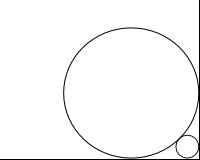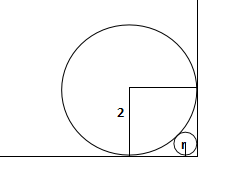Question 28

# A circle with radius 2 is placed against a right angle. Another smaller circle is also placed as shown in the adjoining figure. What is the radius of the smaller circle?SolutionUsing pythagoras we can find diagonal of small square shown in fig. = 2*$$\sqrt2$$ . Now this is equal to 2 + r +  $$\sqrt2$$ *r. Equating we get r = $$6- 4 \sqrt{2}$$

• All Quant CAT Formulas and shortcuts PDF
• 30+ CAT previous papers with solutions PDF

##### Pavan Kumar

1 year, 7 months ago

Excellent way of approach. Brilliant

##### SAHIL KAUSHAL

2 years ago

that Was brilliant

OR